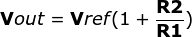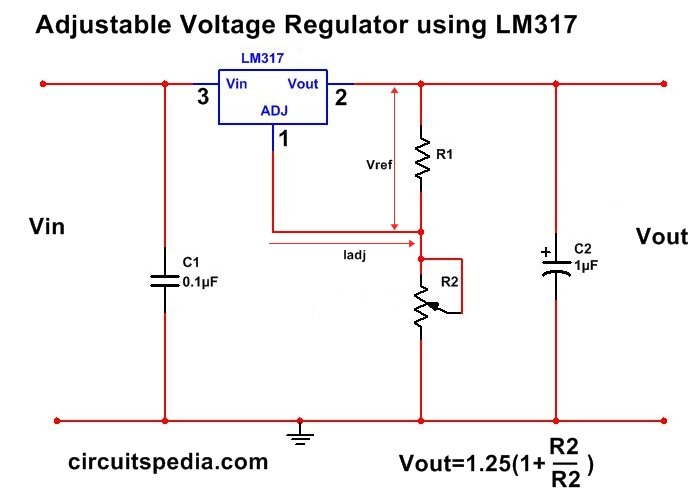1.5 A Adjustable DC Variable Voltage Regulator Using LM317 ic

The LM317T is an adjustable three-terminal positive-voltage regulator. This regulator IC capable of supplying more than 1.5 A over an output-voltage range of 1.25 V to 37 V. To set the output voltage it requires only two external resistors .

Resistors R1 and R2 set the output to any desired voltage over the adjustment range 1.2v to 37v. It has current limiting, thermal overload protection, and safe operating area protection. Overload protection remains functional even if the ADJUST terminal is disconnected. This device can also be used as a programmable output regulator, or by connecting a fixed resistor between the adjustment and output, the LM317 can be used as a precision current regulator.

the LM317 develops and maintains a nominal 1.25 V between its output and adjustment terminals called reference voltage (Vref) . This reference voltage is converted to a programming current (IPROG) by R1 , and this constant current flows through R2 to ground. The output voltage desired can be calculated using.

(IAdj) represents an error Current from the adjustment terminal , With Typical  LM317 IC value
Iadj is to less than 100μ A and keep it constant. Vref= 1.25V. If the load current is less than this minimum, the output voltage will rise. Since the LM317 is a floating regulator, it is only the voltage differential across the circuit which is important to performance, and operation at high voltages with respect to ground is possible.

Iadj is very small amount and it can be ignored in practical applicationExample

Determine the Regulated output voltage of a circuit which haveR1=240 Ω and R2=2.4KΩ

Solution=   We know that Vout= 1.25(1+2.4KΩ/240Ω)

= 13.75V

For 12v output- R1=220Ω, R2=1.8k

For 8v output- R1= 220Ω, R2= 1.2k

For 9v output - R1 = 220Ω, R2 = 1.3k

For 5v output- R1= 220Ω, R2=680Ω

For 3v output- R1=220Ω , R2= 330Ω

Features of LM317

Output Voltage Range Adjustable From 1.25 V to 37 V
Output Current Greater Than 1.5 A
Internal Short-Circuit Current Limiting
Output Safe-Area Compensation

The main disadvantage of LM317 is the voltage is that as much as 2.5 volts is dropped or lost as heat across the regulator. for example, if we need to 9v at output then the input voltage will need to minimum 12 volts or more if the output voltage is to remain stable under maximum load conditions. This voltage drop across the regulator is called “dropout”. A heatsinking is required to keep the regulator cool.